1570868441

# How to build a Neural Network from scratch in Python

## What’s a Neural Network?

Neural Networks are like the workhorses of Deep learning. With enough data and computational power, they can be used to solve most of the problems in deep learning. It is very easy to use a Python or R library to create a neural network and train it on any dataset and get a great accuracy.

Most introductory texts to Neural Networks brings up brain analogies when describing them. Without delving into brain analogies, I find it easier to simply describe Neural Networks as a mathematical function that maps a given input to a desired output.

Neural Networks consist of the following components

• An input layer, x
• An arbitrary amount of hidden layers
• An output layer, ŷ
• A set of weights and biases between each layer, W and b
• A choice of activation function for each hidden layer, σ. In this tutorial, we’ll use a Sigmoid activation function.

The diagram below shows the architecture of a 2-layer Neural Network (note that the input layer is typically excluded when counting the number of layers in a Neural Network)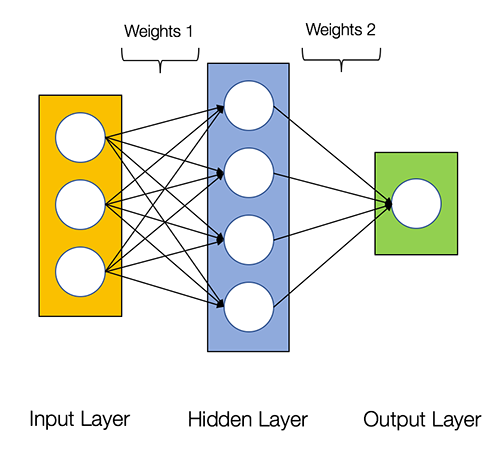Architecture of a 2-layer Neural Network

Creating a Neural Network class in Python is easy.

``````class NeuralNetwork:
def __init__(self, x, y):
self.input      = x
self.weights1   = np.random.rand(self.input.shape,4)
self.weights2   = np.random.rand(4,1)
self.y          = y
self.output     = np.zeros(y.shape)
``````

Training the Neural Network

The output ŷ of a simple 2-layer Neural Network is: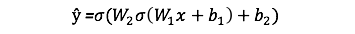You might notice that in the equation above, the weights W and the biases b are the only variables that affects the output ŷ.

Naturally, the right values for the weights and biases determines the strength of the predictions. The process of fine-tuning the weights and biases from the input data is known as training the Neural Network.

Each iteration of the training process consists of the following steps:

• Calculating the predicted output ŷ, known as feedforward
• Updating the weights and biases, known as backpropagation

The sequential graph below illustrates the process.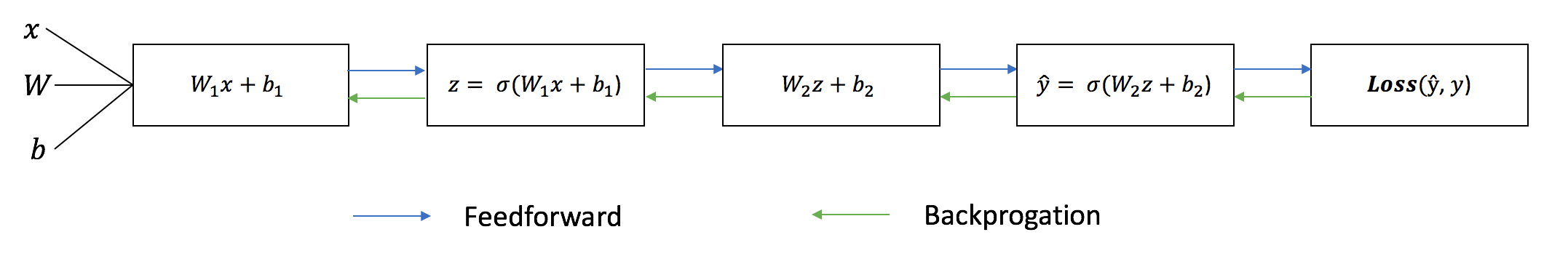### Feedforward

As we’ve seen in the sequential graph above, feedforward is just simple calculus and for a basic 2-layer neural network, the output of the Neural Network is:Let’s add a feedforward function in our python code to do exactly that. Note that for simplicity, we have assumed the biases to be 0.

``````class NeuralNetwork:
def __init__(self, x, y):
self.input      = x
self.weights1   = np.random.rand(self.input.shape,4)
self.weights2   = np.random.rand(4,1)
self.y          = y
self.output     = np.zeros(self.y.shape)

def feedforward(self):
self.layer1 = sigmoid(np.dot(self.input, self.weights1))
self.output = sigmoid(np.dot(self.layer1, self.weights2))
``````

However, we still need a way to evaluate the “goodness” of our predictions (i.e. how far off are our predictions)? The Loss Function allows us to do exactly that.

### Loss Function

There are many available loss functions, and the nature of our problem should dictate our choice of loss function. In this tutorial, we’ll use a simple sum-of-sqaures error as our loss function.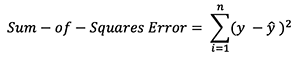That is, the sum-of-squares error is simply the sum of the difference between each predicted value and the actual value. The difference is squared so that we measure the absolute value of the difference.

Our goal in training is to find the best set of weights and biases that minimizes the loss function.

### Backpropagation

Now that we’ve measured the error of our prediction (loss), we need to find a way to propagate the error back, and to update our weights and biases.

In order to know the appropriate amount to adjust the weights and biases by, we need to know the derivative of the loss function with respect to the weights and biases.

Recall from calculus that the derivative of a function is simply the slope of the function.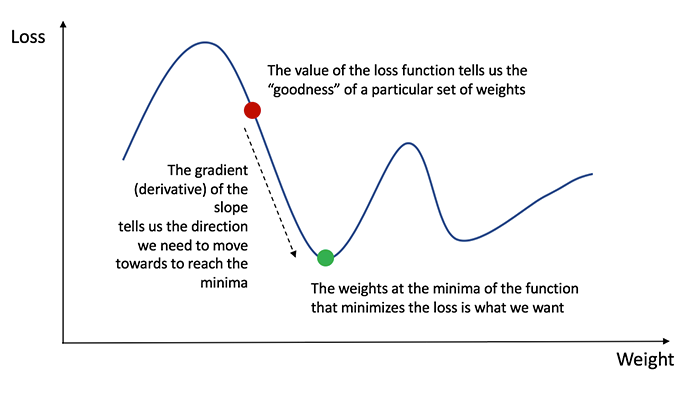If we have the derivative, we can simply update the weights and biases by increasing/reducing with it(refer to the diagram above). This is known as gradient descent.

However, we can’t directly calculate the derivative of the loss function with respect to the weights and biases because the equation of the loss function does not contain the weights and biases. Therefore, we need the chain rule to help us calculate it.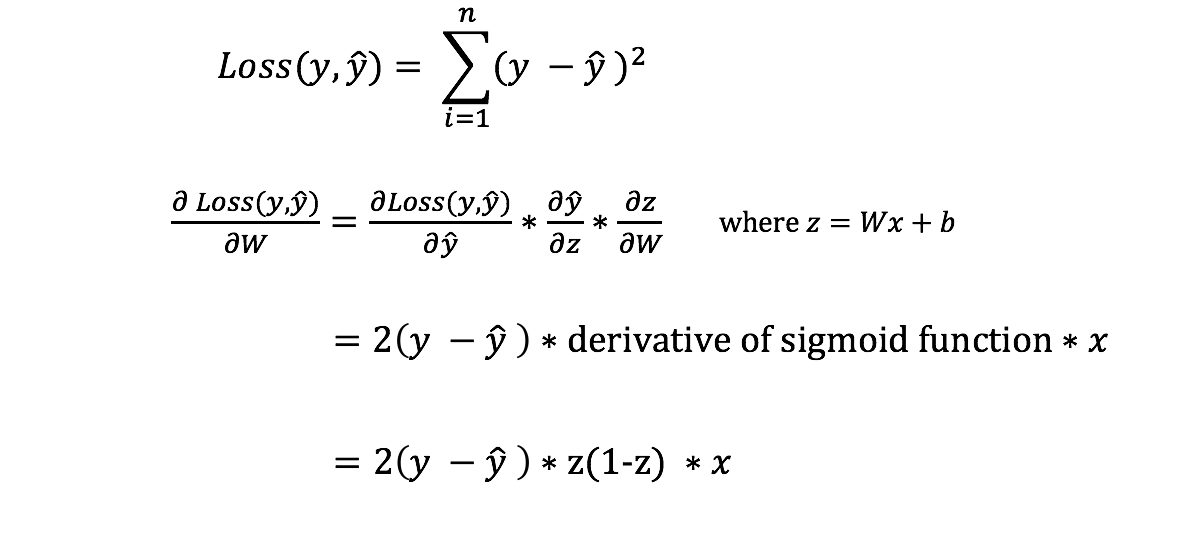Chain rule for calculating derivative of the loss function with respect to the weights. Note that for simplicity, we have only displayed the partial derivative assuming a 1-layer Neural Network.

Phew! That was ugly but it allows us to get what we needed — the derivative (slope) of the loss function with respect to the weights, so that we can adjust the weights accordingly.

Now that we have that, let’s add the backpropagation function into our python code.

``````class NeuralNetwork:
def __init__(self, x, y):
self.input      = x
self.weights1   = np.random.rand(self.input.shape,4)
self.weights2   = np.random.rand(4,1)
self.y          = y
self.output     = np.zeros(self.y.shape)

def feedforward(self):
self.layer1 = sigmoid(np.dot(self.input, self.weights1))
self.output = sigmoid(np.dot(self.layer1, self.weights2))

def backprop(self):
# application of the chain rule to find derivative of the loss function with respect to weights2 and weights1
d_weights2 = np.dot(self.layer1.T, (2*(self.y - self.output) * sigmoid_derivative(self.output)))
d_weights1 = np.dot(self.input.T,  (np.dot(2*(self.y - self.output) * sigmoid_derivative(self.output), self.weights2.T) * sigmoid_derivative(self.layer1)))

# update the weights with the derivative (slope) of the loss function
self.weights1 += d_weights1
self.weights2 += d_weights2
``````

For a deeper understanding of the application of calculus and the chain rule in backpropagation, I strongly recommend this tutorial by 3Blue1Brown.

## Putting it all together

Now that we have our complete python code for doing feedforward and backpropagation, let’s apply our Neural Network on an example and see how well it does.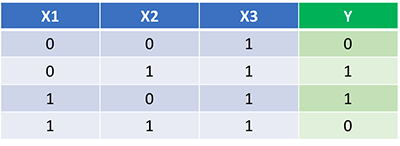Our Neural Network should learn the ideal set of weights to represent this function. Note that it isn’t exactly trivial for us to work out the weights just by inspection alone.

Let’s train the Neural Network for 1500 iterations and see what happens. Looking at the loss per iteration graph below, we can clearly see the loss monotonically decreasing towards a minimum. This is consistent with the gradient descent algorithm that we’ve discussed earlier.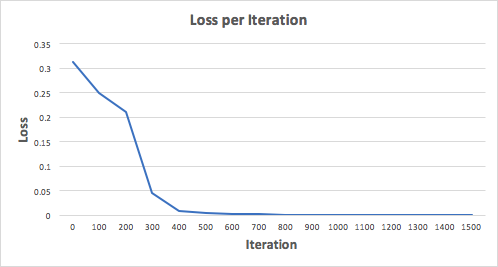Let’s look at the final prediction (output) from the Neural Network after 1500 iterations.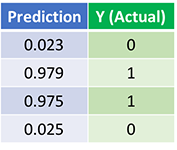Predictions after 1500 training iterations

We did it! Our feedforward and backpropagation algorithm trained the Neural Network successfully and the predictions converged on the true values.

Note that there’s a slight difference between the predictions and the actual values. This is desirable, as it prevents overfitting and allows the Neural Network to generalize better to unseen data.

## What’s Next?

Fortunately for us, our journey isn’t over. There’s still much to learn about Neural Networks and Deep Learning. For example:

• What other activation function can we use besides the Sigmoid function?
• Using a learning rate when training the Neural Network
• Using convolutions for image classification tasks

I’ll be writing more on these topics soon, so do follow me on Medium and keep and eye out for them!

## Final Thoughts

I’ve certainly learnt a lot writing my own Neural Network from scratch.

Although Deep Learning libraries such as TensorFlow and Keras makes it easy to build deep nets without fully understanding the inner workings of a Neural Network, I find that it’s beneficial for aspiring data scientist to gain a deeper understanding of Neural Networks.

This exercise has been a great investment of my time, and I hope that it’ll be useful for you as well!

#deep-learning #python #machine-learning #data-science

## Buddha Community1572021798

thnx a loOoOot!1624752180

## How to build your own Neural Network from scratch in Python

When discussing neural networks, most beginning textbooks create brain analogies. I can define the new neural networks simply as a mathematical function that translates a certain entry to the desired performance without going into brain analogies.

You may note that the weights W and biases b are the only variables in the equation above affecting the output of a given value. The strength of predictions naturally establishes the correct values for weights and biases. The weight and bias adjustment procedure of the input data is known as neural network training.

#neural-networks #artificial-intelligence #python #programming #technology #how to build your own neural network from scratch in python1619510796

## Lambda, Map, Filter functions in python

Welcome to my Blog, In this article, we will learn python lambda function, Map function, and filter function.

Lambda function in python: Lambda is a one line anonymous function and lambda takes any number of arguments but can only have one expression and python lambda syntax is

Syntax: x = lambda arguments : expression

Now i will show you some python lambda function examples:

#python #anonymous function python #filter function in python #lambda #lambda python 3 #map python #python filter #python filter lambda #python lambda #python lambda examples #python map1626775355

## Why use Python for Software Development

No programming language is pretty much as diverse as Python. It enables building cutting edge applications effortlessly. Developers are as yet investigating the full capability of end-to-end Python development services in various areas.

By areas, we mean FinTech, HealthTech, InsureTech, Cybersecurity, and that's just the beginning. These are New Economy areas, and Python has the ability to serve every one of them. The vast majority of them require massive computational abilities. Python's code is dynamic and powerful - equipped for taking care of the heavy traffic and substantial algorithmic capacities.

Programming advancement is multidimensional today. Endeavor programming requires an intelligent application with AI and ML capacities. Shopper based applications require information examination to convey a superior client experience. Netflix, Trello, and Amazon are genuine instances of such applications. Python assists with building them effortlessly.

## 5 Reasons to Utilize Python for Programming Web Apps

Python can do such numerous things that developers can't discover enough reasons to admire it. Python application development isn't restricted to web and enterprise applications. It is exceptionally adaptable and superb for a wide range of uses.

Robust frameworks

Python is known for its tools and frameworks. There's a structure for everything. Django is helpful for building web applications, venture applications, logical applications, and mathematical processing. Flask is another web improvement framework with no conditions.

Web2Py, CherryPy, and Falcon offer incredible capabilities to customize Python development services. A large portion of them are open-source frameworks that allow quick turn of events.

Simple to read and compose

Python has an improved sentence structure - one that is like the English language. New engineers for Python can undoubtedly understand where they stand in the development process. The simplicity of composing allows quick application building.

The motivation behind building Python, as said by its maker Guido Van Rossum, was to empower even beginner engineers to comprehend the programming language. The simple coding likewise permits developers to roll out speedy improvements without getting confused by pointless subtleties.

Utilized by the best

Alright - Python isn't simply one more programming language. It should have something, which is the reason the business giants use it. Furthermore, that too for different purposes. Developers at Google use Python to assemble framework organization systems, parallel information pusher, code audit, testing and QA, and substantially more. Netflix utilizes Python web development services for its recommendation algorithm and media player.

Massive community support

Python has a steadily developing community that offers enormous help. From amateurs to specialists, there's everybody. There are a lot of instructional exercises, documentation, and guides accessible for Python web development solutions.

Today, numerous universities start with Python, adding to the quantity of individuals in the community. Frequently, Python designers team up on various tasks and help each other with algorithmic, utilitarian, and application critical thinking.

Progressive applications

Python is the greatest supporter of data science, Machine Learning, and Artificial Intelligence at any enterprise software development company. Its utilization cases in cutting edge applications are the most compelling motivation for its prosperity. Python is the second most well known tool after R for data analytics.

The simplicity of getting sorted out, overseeing, and visualizing information through unique libraries makes it ideal for data based applications. TensorFlow for neural networks and OpenCV for computer vision are two of Python's most well known use cases for Machine learning applications.

### Summary

Thinking about the advances in programming and innovation, Python is a YES for an assorted scope of utilizations. Game development, web application development services, GUI advancement, ML and AI improvement, Enterprise and customer applications - every one of them uses Python to its full potential.

The disadvantages of Python web improvement arrangements are regularly disregarded by developers and organizations because of the advantages it gives. They focus on quality over speed and performance over blunders. That is the reason it's a good idea to utilize Python for building the applications of the future.

#python development services #python development company #python app development #python development #python in web development #python software development1623135499

## No Code introduction to Neural Networks

### The simple architecture explained

Neural networks have been around for a long time, being developed in the 1960s as a way to simulate neural activity for the development of artificial intelligence systems. However, since then they have developed into a useful analytical tool often used in replace of, or in conjunction with, standard statistical models such as regression or classification as they can be used to predict or more a specific output. The main difference, and advantage, in this regard is that neural networks make no initial assumptions as to the form of the relationship or distribution that underlies the data, meaning they can be more flexible and capture non-standard and non-linear relationships between input and output variables, making them incredibly valuable in todays data rich environment.

In this sense, their use has took over the past decade or so, with the fall in costs and increase in ability of general computing power, the rise of large datasets allowing these models to be trained, and the development of frameworks such as TensforFlow and Keras that have allowed people with sufficient hardware (in some cases this is no longer even an requirement through cloud computing), the correct data and an understanding of a given coding language to implement them. This article therefore seeks to be provide a no code introduction to their architecture and how they work so that their implementation and benefits can be better understood.

Firstly, the way these models work is that there is an input layer, one or more hidden layers and an output layer, each of which are connected by layers of synaptic weights¹. The input layer (X) is used to take in scaled values of the input, usually within a standardised range of 0–1. The hidden layers (Z) are then used to define the relationship between the input and output using weights and activation functions. The output layer (Y) then transforms the results from the hidden layers into the predicted values, often also scaled to be within 0–1. The synaptic weights (W) connecting these layers are used in model training to determine the weights assigned to each input and prediction in order to get the best model fit. Visually, this is represented as:

#machine-learning #python #neural-networks #tensorflow #neural-network-algorithm #no code introduction to neural networks1602968400

## Python Tricks Every Developer Should Know

Python is awesome, it’s one of the easiest languages with simple and intuitive syntax but wait, have you ever thought that there might ways to write your python code simpler?

In this tutorial, you’re going to learn a variety of Python tricks that you can use to write your Python code in a more readable and efficient way like a pro.

### Let’s get started

Swapping value in Python

Instead of creating a temporary variable to hold the value of the one while swapping, you can do this instead

``````>>> FirstName = "kalebu"
>>> LastName = "Jordan"
>>> FirstName, LastName = LastName, FirstName
>>> print(FirstName, LastName)
('Jordan', 'kalebu')
``````

#python #python-programming #python3 #python-tutorials #learn-python #python-tips #python-skills #python-development